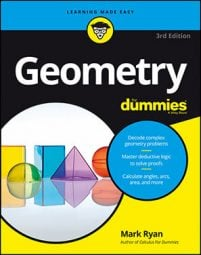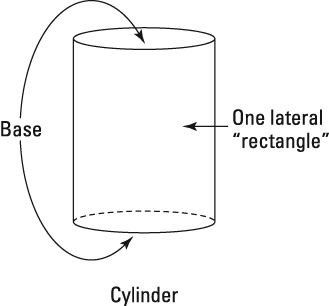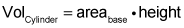##### Geometry For DummiesThe volume of an object is how much space the object takes up — or, if you were to drop the object into a full tub of water, how much water would overflow.

To calculate the volume of a cylinder, you need to know its height and the area of its base. Because a cylinder is a flat-top figure (a solid with two congruent, parallel bases), the base can be either the top or bottom.

If you know a cylinder's height and lateral area, but not its radius, you can use the formula for surface area to find the radius, and then calculate the volume from there.

The lateral area of a cylinder is basically one rectangle rolled into a tube shape. Think of the lateral area of a cylinder as one rectangular paper towel that rolls exactly once around a paper towel roll. The base of this rectangle (you know, the part of the towel that wraps around the bottom of the roll) is the same as the circumference of the cylinder's base. And the height of the paper towel is the same as the height of the cylinder.## Use this formula to calculate the volume of a cylinderNow for a cylinder problem:

To use the volume formula, you need the cylinder's height (which you know) and the area of its base. To get the area of the base, you need its radius. And to get the radius, you can use the surface area formula and solve for r:

Remember that this "rectangle" is rolled around the cylinder and that the "rectangle's" base is the circumference of the cylinder's circular base. You fill in the equation as follows:

Now set the equation equal to zero and factor:

The radius can't be negative, so it's 5. Now you can finish with the volume formula:

That does it.